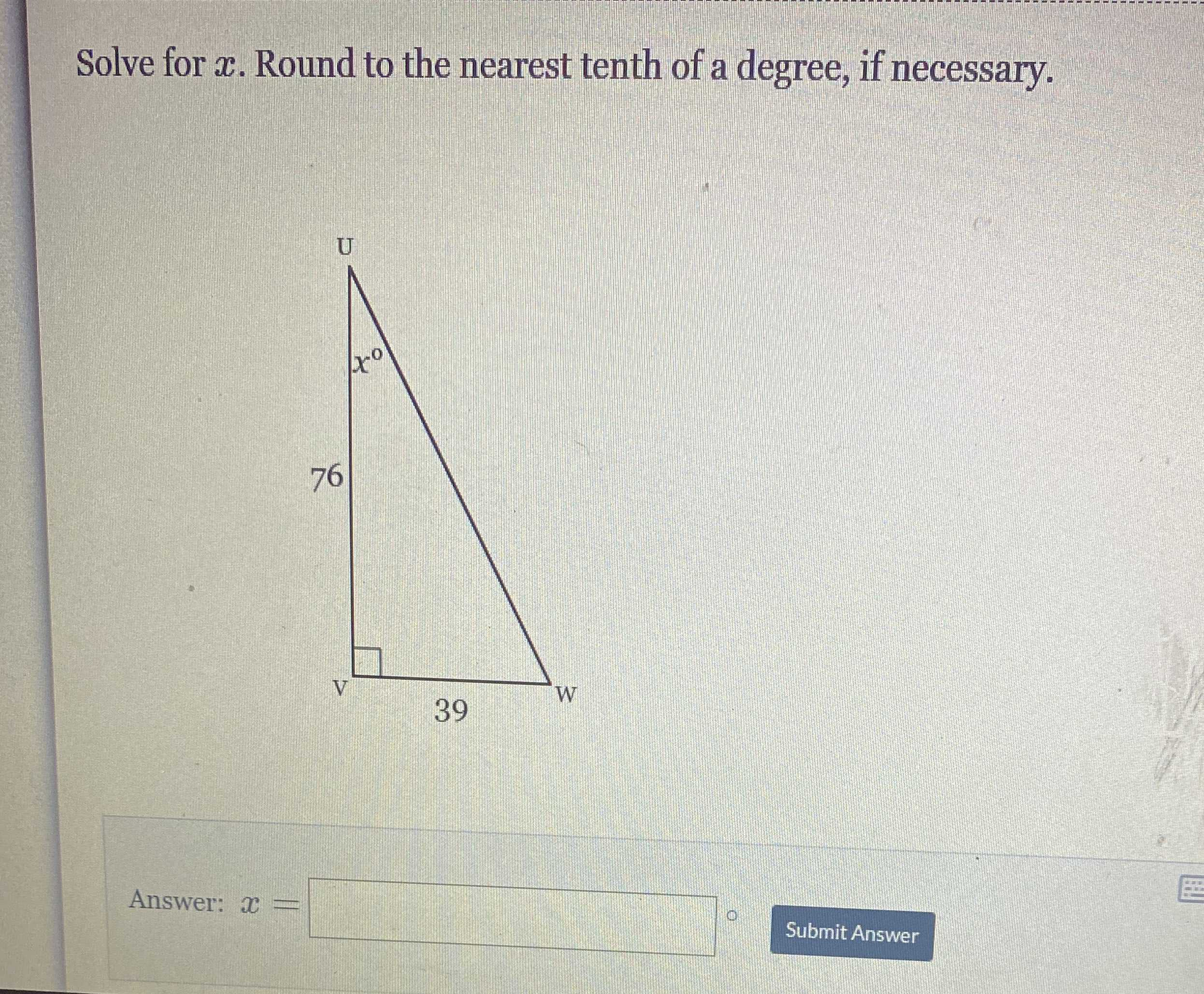### Still have math questions?

Trigonometry
QuestionSolve for $$x$$ . Round to the nearest tenth of a degree, if necessary.

x = tan$$^{- 1}$$(39/76)